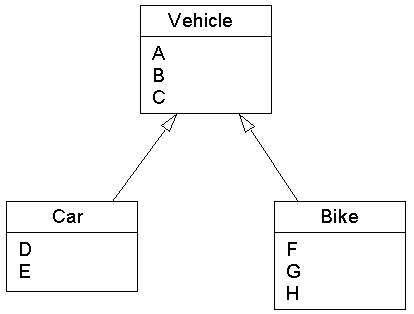Tutorials

# Objective-C Inheritance

Objective-C enables programmer to inherit common methods and properties from other class, known as inheritance. Class from methods and properties are inherited known as Base Class and class that inherits known as Derived Class.

Objective-C enables programmer to inherit common methods and properties from other class, known as inheritance. Class from methods and properties are inherited known as Base Class and class that inherits known as Derived Class.

# Objective-C Inheritance

Objective-C enables programmer to inherit common methods and properties from other class, known as inheritance. Class from methods and properties are inherited known as Base Class and class that inherits known as Derived Class. derived class only specifies how it is different with base class and everything else is taken to be the same. Here in the figure given below Vehicle is the base class and both Car and Bike are derived classes so that these classes can use methods and properties of Vehicle class.Example:

This is code of base class.

FirstClass.h  FirstClass.m

 `#import` ```@interface FirstClass:NSObject { } int num1; -(void)setNum1 :(int) x; -(int)getNum1; @end``` #import "FirstClass.h" @implementation FirstClass   -(void)setNum1 :(int) x {   num1 = x;   printf("num1 is : %d \n", num1);   }   -(int)getNum1 {    return num1;   } @end

This is code of derived class.

SecondClass.h      SecondClass.m

 `#import "FirstClass.m"` ```@interface SecondClass: FirstClass { int num2 ; }``` ```-(void)setNum2 :(int) y; -(int)mul; @end``` ```#import "SecondClass.h" #import "FirstClass.h"``` ```@implementation SecondClass -(id) init { self = [super init]; return self; } -(void)setNum2 :(int) y { num2 = y ; printf("num2 is : %d \n", num2); } -(int)mul { return num2*[self getNum1]; } @end```

This is code of main class.
main.m

 ``` #import "SecondClass.m" #import ``` ```int main() { FirstClass *obj1 = [[FirstClass alloc] init]; SecondClass *obj2 = [[SecondClass alloc] init];``` ``` [obj1 setNum1 : 10 ]; [obj2 setNum2 : 15 ]; printf("Multiplication Result : %d \n",[obj2 mul]); return 0; }```

Output:

 ```num1 is : 10 num2 is : 15 Multiplication Result : 150```